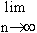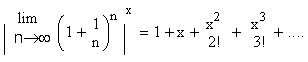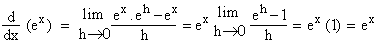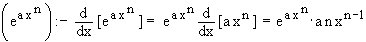Home MonkeyNotes Printable Notes Digital Library Study Guides Study Smart Parents Tips College Planning Test Prep Fun Zone Help / FAQ How to Cite New Title Request

4.12 Derivatives of Exponential and Logarithmic Functions

The number  e Therefore the limit of, as n approaches infinity isThe number defined by, or by the sum of the convergent series, is denoted by  e . Its value, correct to 6 decimal places is 2 . 718282 i.e. clearly 2 < e < 3. It is also a fundamental constant like p and it is the base of the natural or Napierian, or hyperbolic, logarithms.

The exponential function ex which is sometimes written as

e x p x isYour browser does not support the IFRAME tag.

Another important function is* Exponential functions in which a variable quantity occurs as an index, or exponent.

The functionare examples of exponential functions.

Now , let y = f ( x ) = ex then f ( x + h ) = e x + h = ex . eh

Then by the 1st principal of derivatives we getDifferentiation ofDifferentiation of log x:-

Let y = logyex i.e. f ( x ) = log x Þ f ( x + h ) = log ( x + h )

Index

4. 1 Derivability At A Point
4. 2 Derivability In An Interval
4. 3 Derivability And Continuity Of A Function At A Point
4. 4 Some Counter Examples

4. 5 Interpretation Of Derivatives
4. 6 Theorems On Derivatives (differentiation Rules)
4. 7 Derivatives Of Standard Functions
4. 8 Derivative Of A Composite Function
4. 9 Differentiation Of Implicit Functions

4.10 Derivative Of An Inverse Function
4.11 Derivatives Of Inverse Trigonometric Functions
4.12 Derivatives Of Exponential & Logarithmic Functions
4.13 Logarithmic Differentiation
4.14 Derivatives Of Functions In Parametric Form
4.15 Higher order Derivatives

Chapter 5